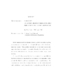## A GRID-FREE LAGRANGIAN DILATATION ELEMENT METHOD WITH APPLICATION TO COMPRESSIBLE FLOW##### Files
umi-umd-1976.pdf(4.38 MB)
In the computational fluid dynamics research, grid-free methods are getting more attention as an alternative to traditional grid-based methods due to two important reasons. First, grid-free methods can be very easily adapted into applications involving complicated geometries. Secondly, they are less vulnerable to numerical diffusion introduced by spatial discretization than in grid-based schemes. A new grid-free Lagrangian dilatation element method for compressible flow has been developed in this research as an extension of incompressible vortex methods. It differs from grid-based numerical methods in a number of ways. The discretization is represented by a group of Lagrangian particles that are convected with the fluid flow velocities instead of a fixed spatial grid system. The velocity of the flow field, necessary in each time step to move the computational elements, is recovered from the dilatation distribution similar to the 'Biot-Savart' law used in incompressible vortex methods. The Fast Multi-pole Method (FMM) is used to speed up the process and reduce the cost from $O(N^2)$ down to $O(N\log N)$. Each computational particle carries physical properties such as dilatation, temperature, density and geometric volume. These properties are governed by the Lagrangian governing equations derived from the Navier-Stokes equations. While the computational elements are convected in the flow, their properties are updated by integrating their corresponding governing equations. The spatial derivatives appearing in the Lagrangian governing equations are evaluated by using moving least-square fitting. The implementation of several different boundary conditions has been developed in this research. The non-penetration wall boundary condition is implemented by adding a potential velocity field to that recovered from the dilatation elements so as to cancel the normal component at the wall. The zero-gradient of properties at the wall such as temperature and density is enforced by a technique called particle reflection. The inflow and outflow conditions are implemented with the help of the characteristic waves moving up and down-stream. The addition and removal of Lagrangian computational elements at the inlet and outlet are implemented to ensure that the computational domain is fully covered by an approximately uniform distribution of particles with roughly comparable volumes. The new grid-free dilatation method is applied to the compressible oscillating waves in an enclosed tube and a subsonic nozzle flow. Both one-dimensional and two-dimensional results are shown and compared with either the exact solutions or the solutions given by other proven numerical schemes. Good agreement of these results helps to establish the correctness of the present method. Future work will accommodate viscous terms and shock waves, which is given a brief discussion at the end of this thesis.